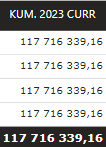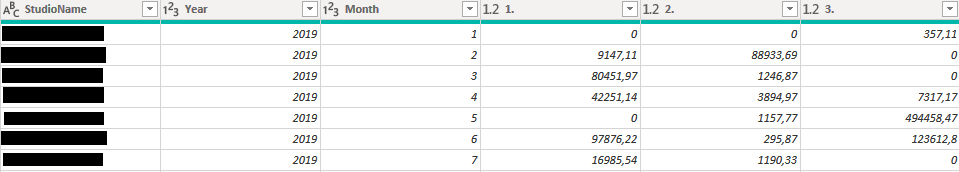cancel
Showing results for
Did you mean:Frequent Visitor

## How do I use my filtered Month in a DAX correctly?

I have a table with Name column, Month column, Year column and Income columns. I will put an image here, but here is column description in text:

The Year and Month columns are just integers. Like: 1 is January in the Month column and 6 is for example June in the Month column.

Income columns are named as days so: 1, 2, 3, 4... and each are a floating point data type with values like 13 470,50 etc.

I have the Year and Month set as my page filter. For example Month = 4 and Year = 2023.

I am trying to write a DAX that takes the Month of the filter (So 4 in our example) PLUS ALL THE MONTHS PRIOR (So Month 1 + Month 2 + Month 3 + Month 4) and add up all the incomes in the Income columns.

In other words add up values in the 31 income columns and add that number cumulatively for each month until the month that is in the filter.

What I tried:

KUM. 2023 = VAR currentSelectedYear = SELECTEDVALUE( vwPBI_Forecast[Year]) RETURN - CALCULATE(SUM(vwPBI_Forecast[1.])+SUM(vwPBI_Forecast[2.])+SUM(vwPBI_Forecast[3.])+SUM(vwPBI_Forecast[4.])+SUM(vwPBI_Forecast[5.])+SUM(vwPBI_Forecast[6.])+SUM(vwPBI_Forecast[7.])+SUM(vwPBI_Forecast[8.])+SUM(vwPBI_Forecast[9.])+SUM(vwPBI_Forecast[10.])+SUM(vwPBI_Forecast[11.])+SUM(vwPBI_Forecast[12.])+SUM(vwPBI_Forecast[13.])+SUM(vwPBI_Forecast[14.])+SUM(vwPBI_Forecast[15.])+SUM(vwPBI_Forecast[16.])+SUM(vwPBI_Forecast[17.])+SUM(vwPBI_Forecast[18.])+SUM(vwPBI_Forecast[19.])+SUM(vwPBI_Forecast[20.])+SUM(vwPBI_Forecast[21.])+SUM(vwPBI_Forecast[22.])+SUM(vwPBI_Forecast[23.])+SUM(vwPBI_Forecast[24.])+SUM(vwPBI_Forecast[25.])+SUM(vwPBI_Forecast[26.])+SUM(vwPBI_Forecast[27.])+SUM(vwPBI_Forecast[28.])+SUM(vwPBI_Forecast[29.])+SUM(vwPBI_Forecast[30.])+SUM(vwPBI_Forecast[31.]), ALL(vwPBI_Forecast[Month]), ALL(vwPBI_Forecast[Year]), FILTER ( ALL (vwPBI_Forecast),INT ( vwPBI_Forecast[Year] ) = currentSelectedYear )) *-1

This gives me this result:The rows are the values from Name column. Why are all the rows the same? I need every row to contain the sum for the corresponding Name, not the total in every row.

This is the data set to better illustrate the description above:I know it's probably something minor, but I am at my wit's end. If anyone has a suggestion for me, I will be most grateful.

THANK YOU!Super User

Hi,

In your case here is an example:

RT example =
VAR MaxDate = MAX ( 'Calendar'[Date] ) -- Saves the last visible date
RETURN
CALCULATE (
SUM('Table (30)'[Column2]),            -- Computes sales amount
'Calendar'[Date] <= MaxDate,   -- Where date is before the last visible date
ALL ( 'Calendar' )               -- Removes any other filters from calendar
)

End result:data:I hope this post helps to solve your issue and if it does consider accepting it as a solution and giving the post a thumbs up!

Proud to be a Super User!Announcements#### Exclusive opportunity for Women!

Join us for a free, hands-on Microsoft workshop led by women trainers for women where you will learn how to build a Dashboard in a Day!#### Power Platform Conference-Power BI and Fabric Sessions

Join us Oct 1 - 6 in Las Vegas for the Microsoft Power Platform Conference.Top Solution Authors
Top Kudoed Authors
Users online (3,295)